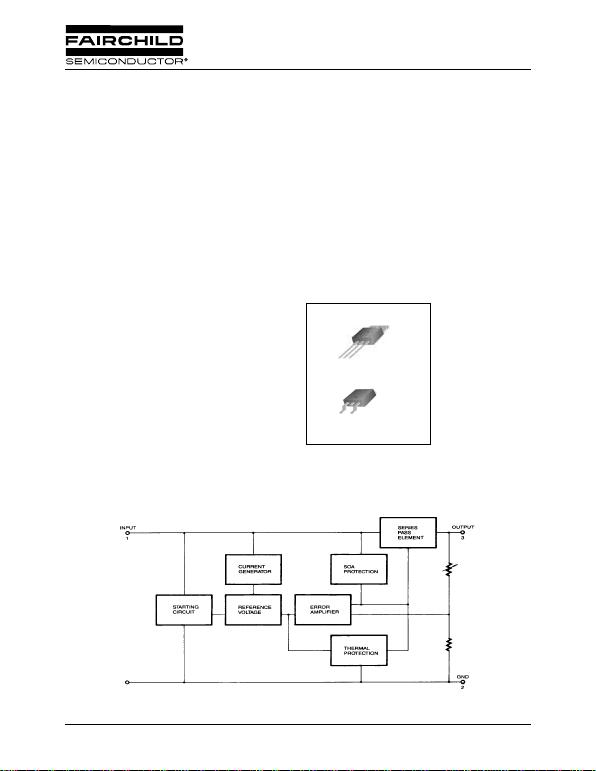www.fairchildsemi.com
Rev. 1.0.1
Features
Output Current up to 1A
Output Voltages of 5, 6, 8, 9, 10, 12, 15, 18, 24V
Short Circuit Protection
Output Transist or Safe Operating Ar ea P r otection
Description
The MC78XX/LM78XX/M C78XXA series of three
terminal positive regulators are available in the
TO-220/D-PAK package and with several fixed output
voltages, making them useful in a wide range of
applications. Each type employs internal current limiting,
thermal shut down and safe operating area protection,
making it essentially indestructible. If adequate heat sinking
is provided, they can deliver over 1A output current.
Although designed primarily as fixed voltage regulators,
these devices can be used with external components to
TO-220
D-PAK
1. Input 2. GND 3. Output
1
1
Internal Block Digram
MC78XX/LM78XX/MC78XXA
3-Terminal 1A Positive Voltage Regulator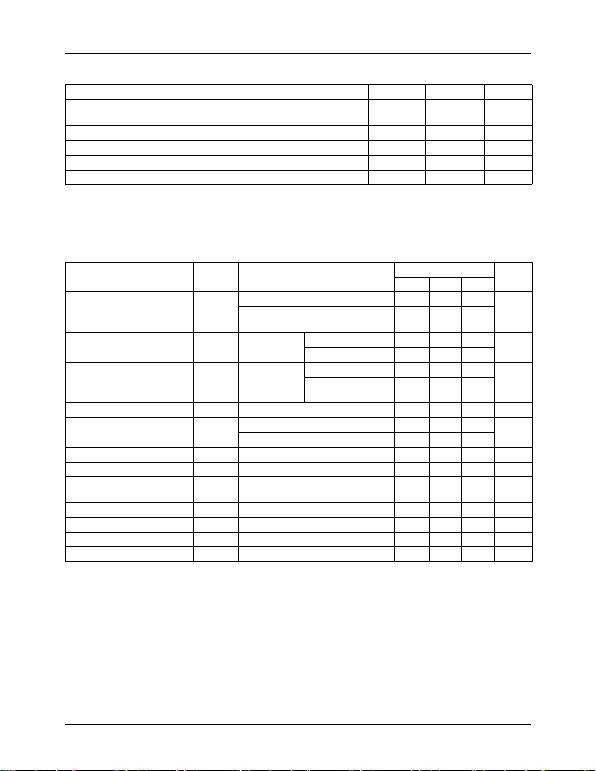MC78XX/LM78XX/MC78XXA
2
Absolute Maximum Ratings
Electrical Characteristics (MC7805/LM7805)
(Refer to test circuit ,0°C < TJ < 125°C, IO = 500mA, VI = 10V, CI= 0.33µF, CO= 0.1µF, unless otherwise specified)
Note:
1. Load and line regulation are specified at constant junction temperature. Changes in Vo du e to he atin g effe cts mus t be tak en
into account separately. Pulse testing with low duty is used.
Parameter Symbol Value Unit
Input Voltage (for VO = 5V to 18V)
(for VO = 24V) VI
VI35
40 V
V
Thermal Resistance Junction-Cases (TO-220) RθJC 5oC/W
Thermal Resistance Junction-Air (TO-220) RθJA 65 oC/W
Operating Temperature Range TOPR 0 ~ +125 oC
Storage Temperature Range TSTG -65 ~ +150 oC
Parameter Symbol Conditions MC7805/LM7805 Unit
Min. Typ. Max.
Output Voltage VOTJ =+25 oC 4.8 5.0 5.2
5.0mA Io 1.0A, PO 15W
VI = 7V to 20V 4.75 5.0 5.25 V
Line Regulation (Note1) Regline TJ=+25 oCVO = 7V to 25V - 4.0 100 mV
VI = 8V to 12V - 1.6 50
Load Regulation (Note1) Regload TJ=+25 oCIO = 5.0mA to1.5A - 9 100 mV
IO =250mA to
750mA -450
Quiescent Current IQTJ =+25 oC-5.08.0mA
Quiescent Current Change IQIO = 5mA to 1.0A - 0.03 0.5 mA
VI= 7V to 25V - 0.3 1.3
Output Voltage Drift VO/TI
O= 5mA - -0.8 - mV/ oC
Output Noise Voltage VNf = 10Hz to 100KHz, TA=+25 oC-42-µV/Vo
Ripple Rejection RR f = 120Hz
VO = 8V to 18V 62 73 - dB
Dropout Voltage VDrop IO = 1A, TJ =+25 oC-2-V
Output Resistance rOf = 1KHz - 15 - m
Short Circuit Current ISC VI = 35V, TA =+25 oC - 230 - mA
Peak Current IPK TJ =+25 oC-2.2-A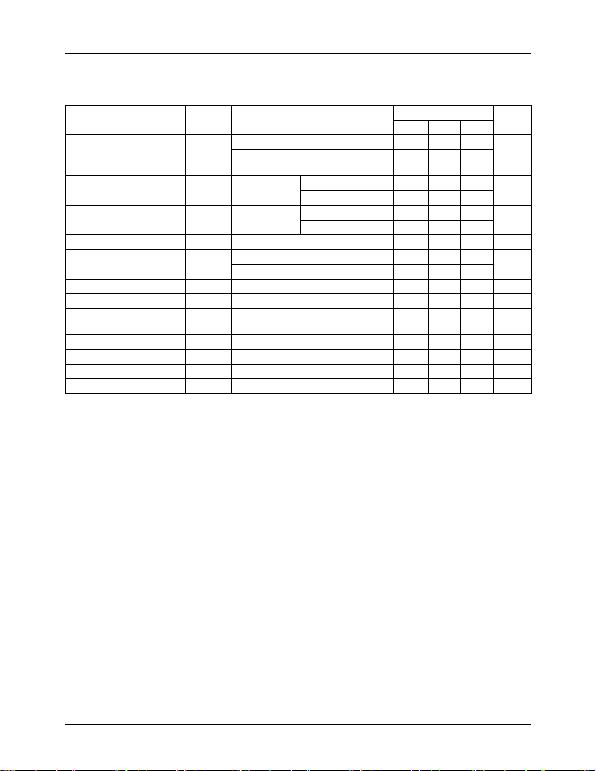MC78XX/LM78XX/MC78XXA
3
Electrical Characteristics (MC7806)
(Refer to test circuit ,0°C < TJ < 125°C, IO = 500mA, VI =11V, CI= 0.33µF, CO= 0.1µF, unless otherwise specified)
Note:
1. Load and line regulation are specified at constant junction temperature. Changes in VO due to hea tin g ef fe ct s mus t be t ak en
into account separately. Pulse testing with low duty is used.
Parameter Symbol Conditions MC7806 Unit
Min. Typ. Max.
Output Voltage VOTJ =+25 oC 5.75 6.0 6.25
5.0mA IO1.0A, PO15W
VI = 8.0V to 21V 5.7 6.0 6.3 V
Line Regulation (Note1) Regline TJ =+25 oCVI = 8V to 25V - 5 120 mV
VI = 9V to 13V - 1.5 60
Load Regulation (Note1) Regload TJ =+25 oCIO =5mA to 1.5A - 9 120 mV
IO =250mA to750A - 3 60
Quiescent Current IQTJ =+25 oC-5.08.0mA
Quiescent Current Change IQIO = 5mA to 1A - - 0.5 mA
VI = 8V to 25V - - 1.3
Output Voltage Drift VO/TI
O = 5mA - -0.8 - mV/ oC
Output Noise Voltage VNf = 10Hz to 100KHz, TA =+25 oC-45-µV/Vo
Ripple Rejection RR f = 120Hz
VI = 9V to 19V 59 75 - dB
Dropout Voltage VDrop IO = 1A, TJ =+25 oC-2-V
Output Resistance rOf = 1KHz - 19 - m
Short Circuit Current ISC VI= 35V, TA=+25 oC - 250 - mA
Peak Current IPK TJ =+25 oC-2.2-A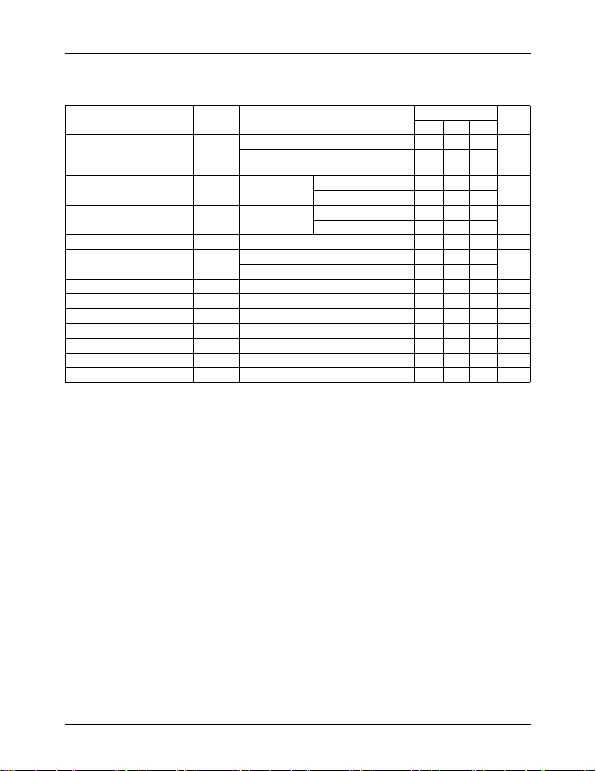MC78XX/LM78XX/MC78XXA
4
Electrical Characteristics (MC7808)
(Refer to test circuit ,0°C < TJ < 125°C, IO = 500mA, VI =14V, CI= 0.33µF, CO= 0.1µF, unless otherwise specified)
Note:
1. Load and line regulation are specified at constant junction temperature. Changes in VO due to he at ing e ffe cts mus t be t ak en
into account separately. Pulse testing with low duty is used.
Parameter Symbol Conditions MC7808 Unit
Min. Typ. Max.
Output Voltage VOTJ =+25 oC 7.7 8.0 8.3
5.0mA IO 1.0A, P O15W
VI = 10.5V to 23V 7.6 8.0 8.4 V
Line Regulation (Note1) Regline TJ =+25 oCVI = 10.5V to 25V - 5.0 160 mV
VI = 11.5V to 17V - 2.0 80
Load Regulation (Note1) Regload TJ =+25 oCIO = 5.0mA to 1.5A - 10 160 mV
IO= 250mA to 750mA - 5.0 80
Quiescent Current IQTJ =+25 oC-5.08.0mA
Quiescent Current Change IQIO = 5mA to 1.0A - 0.05 0.5 mA
VI = 10.5A to 25V - 0.5 1.0
Output Voltage Drift VO/TI
O = 5mA - -0.8 - mV/ oC
Output Noise Voltage VNf = 10Hz to 100KHz, TA =+25 oC-52-µV/Vo
Ripple Rejection RR f = 120Hz, VI= 11.5V to 21.5V 56 73 - dB
Dropout Voltage VDrop IO = 1A, TJ=+25 oC-2-V
Output Resistance rOf = 1KHz - 17 - m
Short Circuit Current ISC VI= 35V, TA =+25 oC - 230 - mA
Peak Current IPK TJ =+25 oC-2.2-A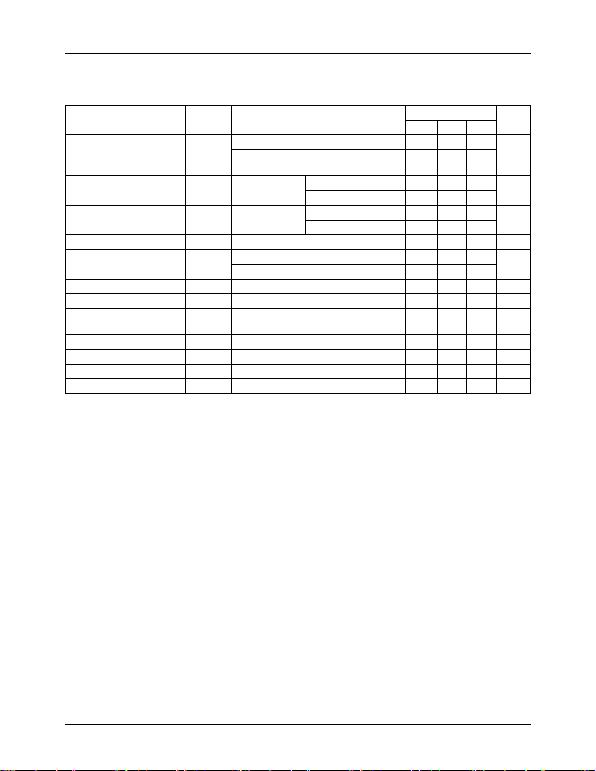MC78XX/LM78XX/MC78XXA
5
Electrical Characteristics (MC7809)
(Refer to test circuit ,0°C < TJ < 125°C, IO = 500mA, VI =15V, CI= 0.33µF, CO= 0.1µF, unless otherwise specified)
Note:
1. Load and line regulation are specified at constant junction temperature. Changes in VO due to hea tin g ef fe ct s mus t be t ak en
into account separately. Pulse testing with low duty is used.
Parameter Symbol Conditions MC7809 Unit
Min. Typ. Max.
Output Voltage VOTJ =+25°C 8.65 9 9.35
5.0mA IO 1.0A, PO 15W
VI= 11.5V to 24V 8.6 9 9.4 V
Line Regulation (Note1) Regline TJ=+25°CVI = 11.5V to 25V - 6 180 mV
VI = 12V to 17V - 2 90
Load Regulation (Note1) Regload TJ=+25°CIO = 5mA to 1.5A - 12 180 mV
IO = 250mA to 750mA - 4 90
Quiescent Current IQTJ=+25°C-5.08.0mA
Quiescent Current Change IQIO = 5mA to 1.0A - - 0.5 mA
VI = 11.5V to 26V - - 1.3
Output Voltage Drift VO/TI
O = 5mA - -1 - mV/ °C
Output Noise Voltage VNf = 10Hz to 100KHz, TA =+25 °C-58-µV/Vo
Ripple Rejection RR f = 120Hz
VI = 13V to 23V 56 71 - dB
Dropout Voltage VDrop IO = 1A, TJ=+25°C-2-V
Output Resistance rOf = 1KHz - 17 - m
Short Circuit Current ISC VI= 35V, TA =+25°C - 250 - mA
Peak Current IPK TJ= +25°C-2.2-A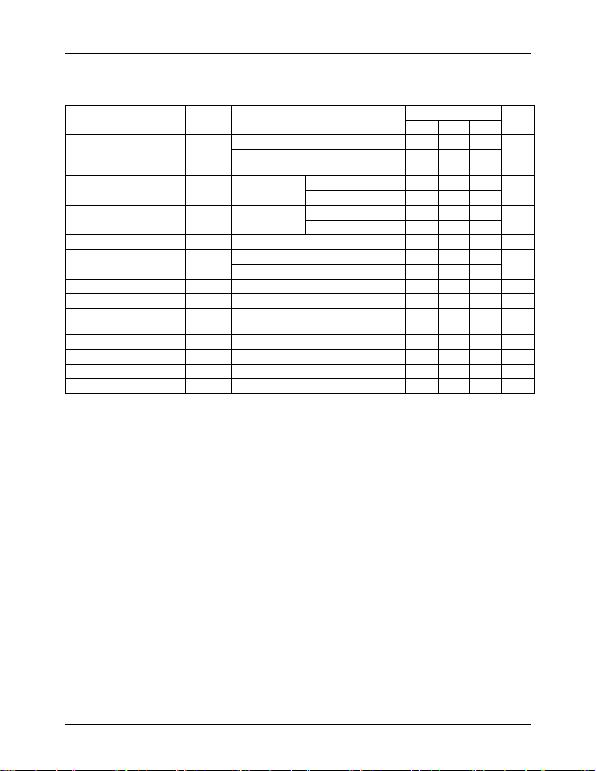MC78XX/LM78XX/MC78XXA
6
Electrical Characteristics (MC7810)
(Refer to test circuit ,0°C< TJ < 125°C, IO = 500mA, VI =16V, CI= 0.33µF, CO=0.1µF, unless otherwise specified)
Note:
1. Load and line regulation are specified at constant junction temperature. Changes in VO due to he at ing e ffe cts mus t be t ak en
into account separately. Pulse testing with low duty is used.
Parameter Symbol Conditions MC7810 Unit
Min. Typ. Max.
Output Voltage VOTJ =+25 °C 9.6 10 10.4
5.0mA IO1.0A, PO 15W
VI = 12.5V to 25V 9.5 10 10.5 V
Line Regulation (Note1) Regline TJ =+25°CVI = 12.5V to 25V - 10 200 mV
VI = 13V to 25V - 3 100
Load Regulation (Note1) Regload TJ =+25°CIO = 5mA to 1.5A - 12 200 mV
IO = 250mA to 750mA - 4 400
Quiescent Current IQTJ =+25°C-5.18.0mA
Quiescent Current Change IQIO = 5mA to 1.0A - - 0.5 mA
VI = 12.5V to 29V - - 1.0
Output Voltage Drift VO/TI
O = 5mA - -1 - mV/°C
Output Noise Voltage VNf = 10Hz to 100KHz, TA =+25 °C-58-µV/Vo
Ripple Rejection RR f = 120Hz
VI = 13V to 23V 56 71 - dB
Dropout Voltage VDrop IO = 1A, TJ=+25 °C-2-V
Output Resistance rOf = 1KHz - 17 - m
Short Circuit Current ISC VI = 35V, TA=+25 °C - 250 - mA
Peak Current IPK TJ =+25 °C-2.2-A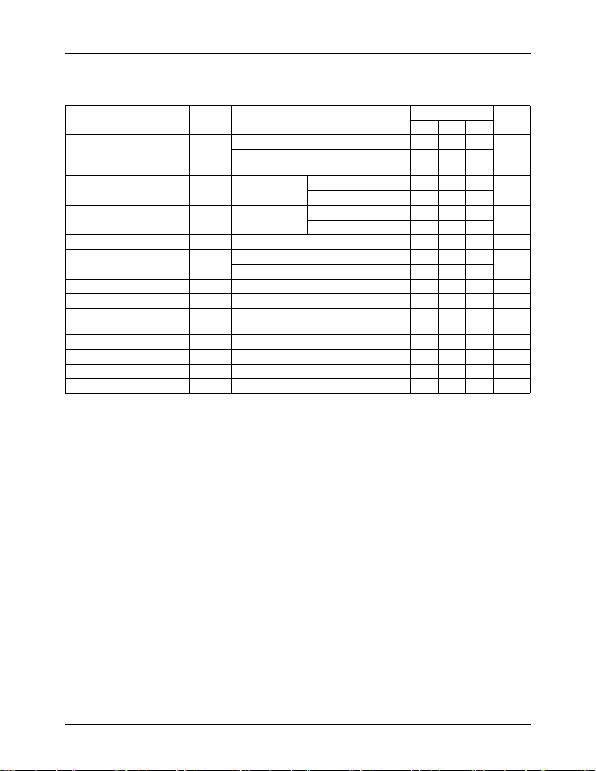MC78XX/LM78XX/MC78XXA
7
Electrical Characteristics (MC7812)
(Refer to test circuit ,0°C < TJ < 125°C, IO = 500mA, VI =19V, CI= 0.33µF, CO=0.1µF, unless otherwise specified)
Note:
1. Load and line regulation are specified at constant junction temperature. Changes in VO due to he at ing e ffe cts mus t be t ak en
into account separately. Pulse testing with low duty is used.
Parameter Symbol Conditions MC7812 Unit
Min. Typ. Max.
Output Voltage VOTJ =+25 oC 11.5 12 12.5
5.0mA IO1.0A, PO15W
VI = 14.5V to 27V 11.4 12 12.6 V
Line Regulation (Note1) Regline TJ =+25 oCVI = 14.5V to 30V - 10 240 mV
VI = 16V to 22V - 3.0 120
Load Regulation (Note1) Regload TJ =+25 oCIO = 5mA to 1.5A - 11 240 mV
IO = 250mA to 750mA - 5.0 120
Quiescent Current IQTJ =+25 oC-5.18.0mA
Quiescent Current Change IQIO = 5mA to 1.0A - 0.1 0.5 mA
VI = 14.5V to 30V - 0.5 1.0
Output Voltage Drift VO/TI
O = 5mA - -1 - mV/ oC
Output Noise Voltage VNf = 10Hz to 100KHz , TA =+25 oC-76-µV/Vo
Ripple Rejection RR f = 120Hz
VI = 15V to 25V 55 71 - dB
Dropout Voltage VDrop IO = 1A, TJ=+25 oC-2-V
Output Resistance rOf = 1KHz - 18 - m
Short Circuit Current ISC VI = 35V, TA=+25 oC - 230 - mA
Peak Current IPK TJ = +25 oC-2.2-A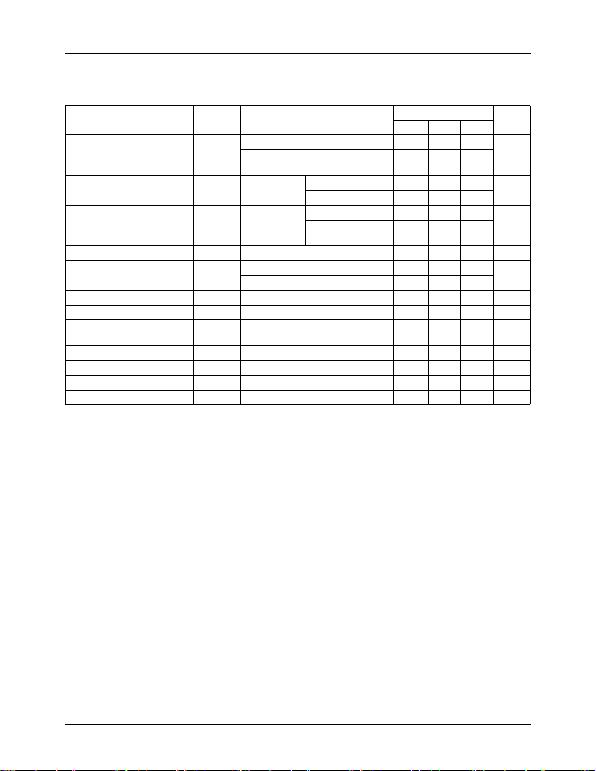MC78XX/LM78XX/MC78XXA
8
Electrical Characteristics (MC7815)
(Refer to test circuit ,0°C < TJ < 125°C, IO = 500mA, VI =23V, CI= 0.33µF, CO=0.1µF, unless otherwise specified)
Note:
1. Load and line regulation are specified at constant junction temperature. Changes in VO due to hea tin g ef fe ct s mus t be t ak en
into account separately. Pulse testing with low duty is used.
Parameter Symbol Conditions MC7815 Unit
Min. Typ. Max.
Output Voltage VOTJ =+25 oC 14.4 15 15.6
5.0mA IO 1.0A, PO 15W
VI = 17.5V to 30V 14.25 15 15.75 V
Line Regulation (Note1) Regline TJ =+25 oCVI = 17.5V to 30V - 1 1 300 mV
VI = 20V to 26V - 3 150
Load Regulation (Note1) Regload TJ =+25 oCIO = 5mA to 1.5A - 12 300 mV
IO = 250mA to
750mA - 4 150
Quiescent Current IQTJ =+25 oC-5.28.0mA
Quiescent Current Change IQIO = 5mA to 1.0A - - 0.5 mA
VI = 17.5V to 30V - - 1.0
Output Voltage Drift VO/TI
O = 5mA - -1 - mV/ oC
Output Noise Voltage VNf = 10Hz to 100KHz , TA =+25 oC-90-µV/Vo
Ripple Rejection RR f = 120Hz
VI = 18.5V to 28.5V 54 70 - dB
Dropout Voltage VDrop IO = 1A, TJ=+25 oC-2-V
Output Resistance rOf = 1KHz - 19 - m
Short Circuit Current ISC VI = 35V, TA=+25 oC - 250 - mA
Peak Current IPK TJ =+25 oC-2.2-A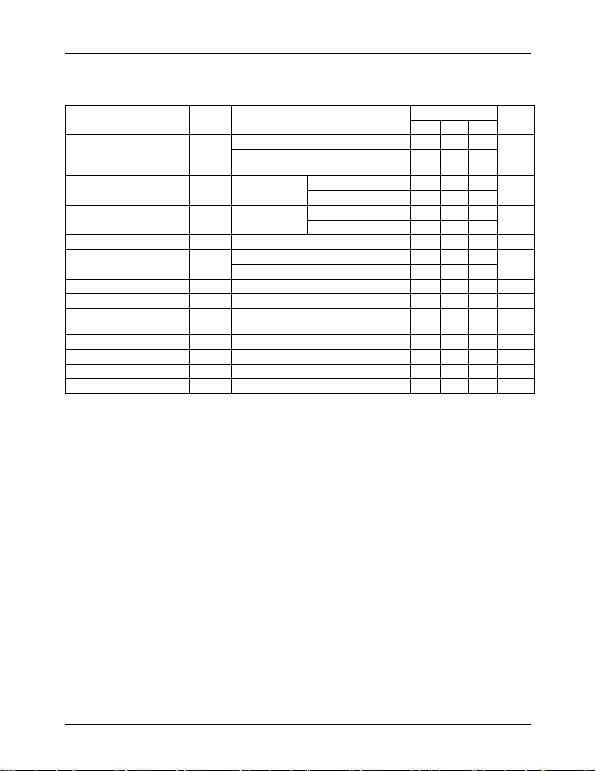MC78XX/LM78XX/MC78XXA
9
Electrical Characteristics (MC7818)
(Refer to test circuit ,0°C < TJ < 125°C, IO = 500mA, VI =27V, CI= 0.33µF, CO=0.1µF, unless otherwise specified)
Note:
1. Load and line regulation are specified at constant junction temperature. Changes in VO due to he at ing e ffe cts mus t be t ak en
into account separately. Pulse testing with low duty is used.
Parameter Symbol Conditions MC7818 Unit
Min. Typ. Max.
Output Voltage VOTJ =+25 oC 17.3 18 18.7
5.0mA IO 1.0A, PO 15W
VI = 21V to 33V 17.1 18 18.9 V
Line Regulation (Note1) Regline TJ =+25 oCVI = 21V to 33V - 15 360 mV
VI = 24V to 30V - 5 180
Load Regulation (Note1) Regload TJ =+25 oCIO = 5mA to 1.5A - 15 360 mV
IO = 250mA to 750mA - 5.0 180
Quiescent Current IQTJ =+25 oC-5.28.0mA
Quiescent Current Change IQIO = 5mA to 1.0A - - 0.5 mA
VI = 21V to 33V - - 1
Output Voltage Drift VO/TI
O = 5mA - -1 - mV/ oC
Output Noise Voltage VNf = 10Hz to 100KHz , TA =+25 oC-110-µV/Vo
Ripple Rejection RR f = 120Hz
VI = 22V to 32V 53 69 - dB
Dropout Voltage VDrop IO = 1A, TJ=+25 oC - 2 - V
Output Resistance rOf = 1KHz - 22 - m
Short Circuit Current ISC VI = 35V, TA=+25 oC - 250 - mA
Peak Current IPK TJ =+25 oC-2.2-A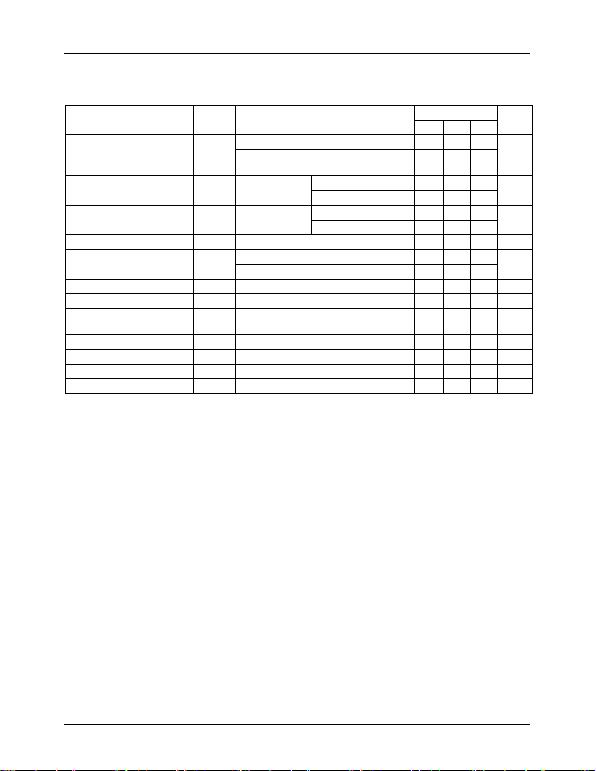MC78XX/LM78XX/MC78XXA
10
Electrical Characteristics (MC7824)
(Refer to test circuit ,0°C < TJ < 125°C, IO = 500mA, VI =33V, CI= 0.33µF, CO=0.1µF, unless otherwise specified)
Note:
1. Load and line regulation are specified at constant junction temperature. Changes in VO due to heating effects must be taken
into account separately. Pulse testing with low duty is used.
Parameter Symbol Conditions MC7824 Unit
Min. Typ. Max.
Output Voltage VOTJ =+25 oC232425
5.0mA IO 1.0A, PO 15W
VI = 27V to 38V 22.8 24 25.25 V
Line Regulation (Note1) Regline TJ =+25 oCVI = 27V to 38V - 17 480 mV
VI = 30V to 36V - 6 240
Load Regulation (Note1) Regload TJ =+25 oCIO = 5mA to 1.5A - 15 480 mV
IO = 250mA to 750mA - 5.0 240
Quiescent Current IQTJ =+25 oC-5.28.0mA
Quiescent Current Change IQIO = 5mA to 1.0A - 0.1 0.5 mA
VI = 27V to 38V - 0.5 1
Output Voltage Drift VO/TI
O = 5mA - -1.5 - mV/ oC
Output Noise Voltage VNf = 10Hz to 100KHz , TA =+25 oC-60-µV/Vo
Ripple Rejection RR f = 120Hz
VI = 28V to 38V 50 67 - dB
Dropout Voltage VDrop IO = 1A, TJ=+25 oC-2-V
Output Resistance rOf = 1KHz - 28 - m
Short Circuit Current ISC VI = 35V, TA=+25 oC - 230 - mA
Peak Current IPK TJ =+25 oC-2.2-A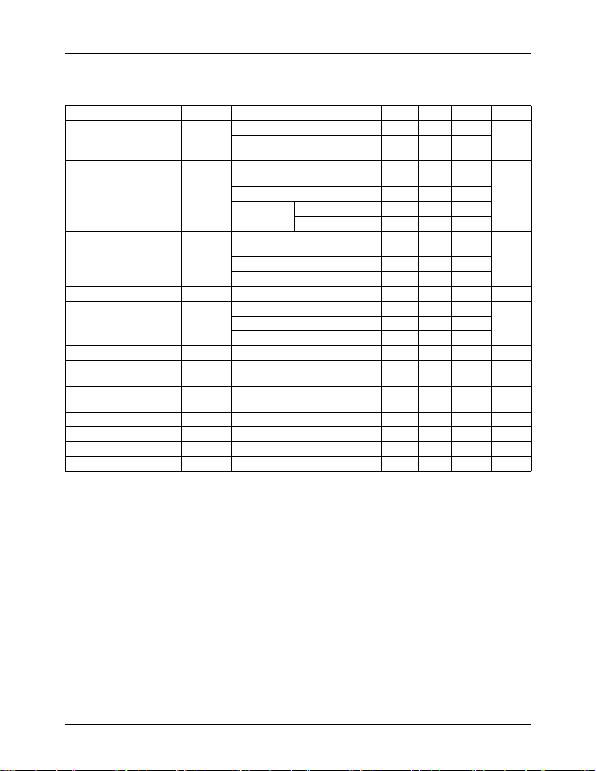MC78XX/LM78XX/MC78XXA
11
Electrical Characteristics (MC7805A)
(Refer to the test circuits. 0°C < TJ < 125°C, Io =1A, V I = 10V, C I=0.33µF, C O=0.1µF, unless otherwise specified)
Note:
1. Load and line regulation are specified at constant junction temperature. Change in VO due to heating effects must be taken
into account separately. Pulse testing with low duty is used.
Parameter Symbol Conditions Min. Typ. Max. Unit
Output Voltage VOTJ =+25 oC4.955.1
V
IO = 5mA to 1A, PO 15W
VI = 7.5V to 20V 4.8 5 5.2
Line Regulation (Note1) Regline
VI = 7.5V to 25V
IO = 500mA -550
mV
VI = 8V to 12V - 3 50
TJ =+25 oCVI= 7.3V to 20V - 5 50
VI= 8V to 12V - 1.5 25
TJ =+25 oC
IO = 5mA to 1.5A - 9 100 mV
IO = 5mA to 1A - 9 100
IO = 250mA to 750mA - 4 50
Quiescent Current IQTJ =+25 oC-5.06mA
Quiescent Current
Change IQ
IO = 5mA to 1A - - 0.5 mAVI = 8 V to 25V, IO = 500mA - - 0.8
VI = 7.5V to 20V, TJ =+25 oC--0.8
Output Voltage Drift V/T Io = 5mA - -0.8 - mV/ oC
Output Noise Voltage VNf = 10Hz to 100KHz
TA =+25 oC-10-µV/Vo
Ripple Rejection RR f = 120Hz, IO = 500mA
VI = 8V to 18V -68- dB
Dropout Voltage VDrop IO = 1A, TJ =+25 oC-2-V
Output Resistance rOf = 1KHz - 17 - m
Short Circuit Current ISC VI= 35V, TA =+25 oC - 250 - mA
Peak Current IPK TJ= +25 oC-2.2-A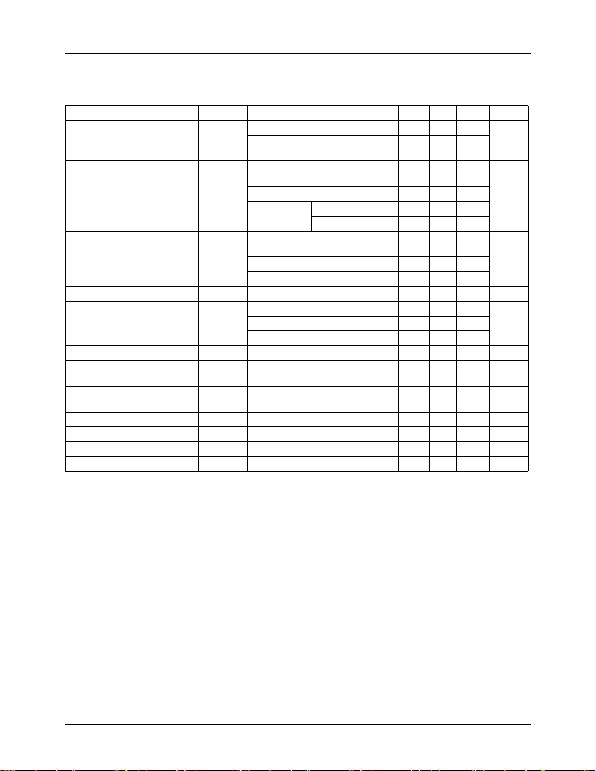MC78XX/LM78XX/MC78XXA
12
Electrical Characteristics (MC7806A)
(Refer to the test circuits. 0°C < TJ < 125°C, Io =1A, V I =11V, C I=0.33µF, C O=0.1µF, unless otherwise specified)
Note:
1. L oad and line regu lat ion a re s pec ifie d at c ons tant junc ti on tem per atur e. Ch ange in VO due to heating effects must be taken
into account separately. Pulse testing with low duty is used.
Parameter Symbol Conditions Min. Typ. Max. Unit
Output Voltage V OTJ =+25 oC 5.58 6 6.12 V
IO = 5mA to 1A, PO 15W
VI = 8.6V to 21V 5.76 6 6.24
Line Regulation (Note1) Regline
VI= 8.6V to 25V
IO = 500mA -560
mV
VI= 9V to 13V - 3 60
TJ =+25 oCVI= 8.3V to 21V - 5 60
VI= 9V to 13V - 1.5 30
TJ =+25 oC
IO = 5mA to 1.5A - 9 100 mV
IO = 5mA to 1A - 4 100
IO = 250mA to 750mA - 5.0 50
Quiescent Current IQTJ =+25 oC-4.36mA
Quiescent Current Change IQ
IO = 5mA to 1A - - 0.5 mAVI = 9V to 25V, IO = 500mA - - 0.8
VI= 8.5V to 21V, TJ =+25 oC--0.8
Output Voltage Drift V/TI
O = 5mA - -0.8 - mV/ oC
Output Noise Voltage VNf = 10Hz to 100KHz
TA =+25 oC-10-µV/V o
Ripple Rejection RR f = 120Hz, IO = 500mA
VI = 9V to 19V -65- dB
Dropout Voltage VDrop IO = 1A, TJ =+25 oC-2-V
Output Resistance rOf = 1KHz - 17 - m
Short Circuit Current ISC VI= 35V, TA =+25 oC - 250 - mA
Peak Current IPK TJ=+25 oC-2.2-A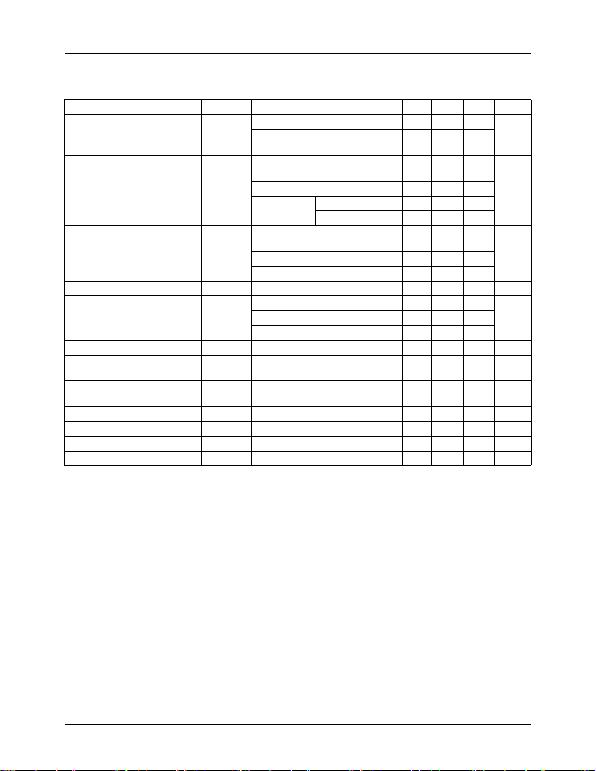MC78XX/LM78XX/MC78XXA
13
Electrical Characteristics (MC7808A)
(Refer to the test circuits. 0°C < TJ < 125°C, Io =1A, V I = 14V, C I=0.33µF, C O=0.1µF, unless otherwise specified)
Note:
1. Load and line regulation are specified at constant junction temperature. Change in VO due to heating effects must be taken
into account separately. Pulse testing with low duty is used.
Parameter Symbol Conditions Min. Typ. Max. Unit
Output Voltage VOTJ =+25 oC 7.84 8 8.16 V
IO = 5mA to 1A, PO 15W
VI = 10.6V to 23V 7.7 8 8.3
Line Regulation (Note1) Regline
VI= 10.6V to 25V
IO = 500mA -680
mV
VI= 11V to 17V - 3 80
TJ =+25 oCVI= 10.4V to 23V - 6 80
VI= 11V to 17V - 2 40
TJ =+25 oC
IO = 5mA to 1.5A - 12 100 mV
IO = 5mA to 1A - 12 100
IO = 250mA to 750mA - 5 50
Quiescent Current IQTJ =+25 oC-5.06mA
Quiescent Current Change IQ
IO = 5mA to 1A - - 0.5 mAVI = 11V to 25V, IO = 500mA - - 0.8
VI= 10.6V to 23V, TJ =+25 oC--0.8
Output Voltage Drift V/TI
O = 5mA - -0.8 - mV/ oC
Output Noise Voltage VNf = 10Hz to 100KHz
TA =+25 oC-10-µV/Vo
Ripple Rejection RR f = 120Hz, IO = 500mA
VI = 11.5V to 21.5V -62- dB
Dropout Voltage VDrop IO = 1A, TJ =+25 oC-2-V
Output Resistance rOf = 1KHz - 18 - m
Short Circuit Current ISC VI= 35V, TA =+25 oC - 250 - mA
Peak Current IPK TJ=+25 oC-2.2-A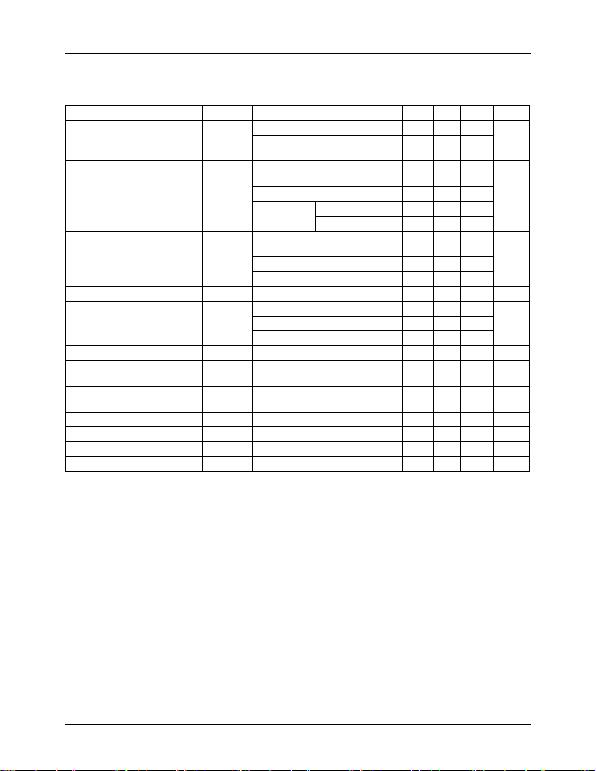MC78XX/LM78XX/MC78XXA
14
Electrical Characteristics (MC7809A)
(Refer to the test circuits. 0°C < TJ < 125°C, Io =1A, V I = 15V, C I=0.33µF, C O=0.1µF, unless otherwise specified)
Note:
1. Load and line regulation are specified at constant, junction temperature. Change in VO due to heating effects must be taken
into account separately. Pulse testing with low duty is used.
Parameter Symbol Conditions Min. Typ. Max. Unit
Output Voltage VOTJ =+25°C 8.82 9.0 9.18 V
IO = 5mA to 1A, PO15W
VI = 11.2V to 24V 8.65 9.0 9.35
Line Regulation (Note1) Regline
VI= 11.7V to 25V
IO = 500mA -690
mV
VI= 12.5V to 19V - 4 45
TJ =+25°C VI= 11.5V to 24V - 6 90
VI= 12.5V to 19V - 2 45
TJ =+25°C
IO = 5mA to 1.0A - 12 100 mV
IO = 5mA to 1.0A - 12 100
IO = 250mA to 750mA - 5 50
Quiescent Current IQTJ =+25 °C-5.06.0mA
Quiescent Current Change IQ
VI = 11.7V to 25V, TJ=+25 °C--0.8
mAVI = 12V to 25V, IO = 500mA - - 0.8
IO = 5mA to 1.0A - - 0.5
Output Voltage Drift V/TI
O = 5mA - -1.0 - mV/ °C
Output Noise Voltage VNf = 10Hz to 100KHz
TA =+25 °C-10-µV/V o
Ripple Rejection RR f = 120Hz, IO = 500mA
VI = 12V to 22V -62- dB
Dropout Voltage VDrop IO = 1A, TJ =+25 °C-2.0-V
Output Resistance rOf = 1KHz - 17 - m
Short Circuit Current ISC VI= 35V, TA =+25 °C - 250 - mA
Peak Current IPK TJ=+25°C-2.2-A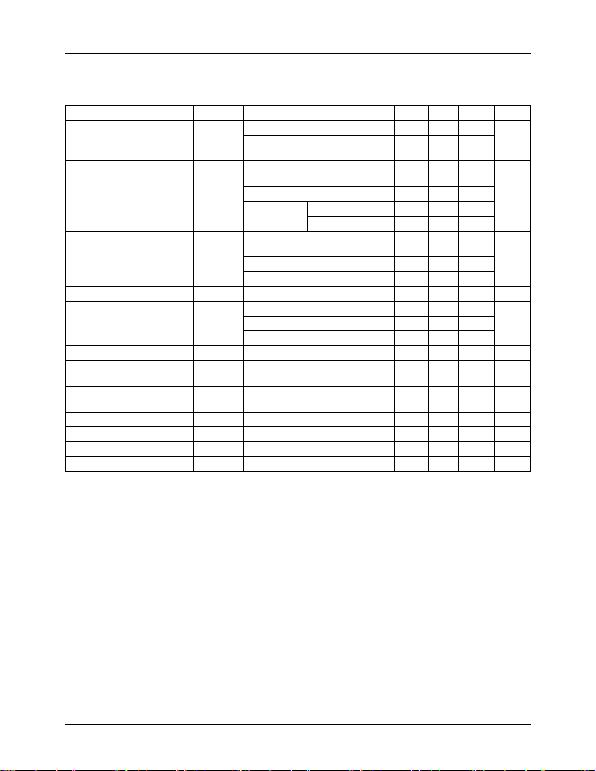MC78XX/LM78XX/MC78XXA
15
Electrical Characteristics (MC7810A)
(Refer to the test circuits. 0°C < TJ < 125°C, Io =1A, V I = 16V, C I=0.33µF, C O=0.1µF, unless otherwise specified)
Note:
1. Load and line regulation are specified at constant junction temperature. Change in VO due to heating effects must be taken
into account separately. Pulse testing with low duty is used.
Parameter Symbol Conditions Min. Typ. Max. Unit
Output Voltage VO TJ =+25°C 9.8 10 10.2 V
IO = 5mA to 1A, PO 15W
VI =12.8V to 25V 9.6 10 10.4
Line Regulation (Note1) Regline
VI= 12.8V to 26V
IO = 500mA - 8 100
mV
VI= 13V to 20V - 4 50
TJ =+25 °C VI= 12.5V to 25V - 8 100
VI= 13V to 20V - 3 50
TJ =+25 °C
IO = 5mA to 1.5A - 12 100 mV
IO = 5mA to 1.0A - 12 100
IO = 250mA to 750mA - 5 50
Quiescent Current IQ TJ =+25 °C-5.06.0mA
Quiescent Current Change IQ
VI = 13V to 26V, TJ=+25 °C--0.5
mA VI = 12.8V to 25V, IO = 500mA - - 0.8
IO = 5mA to 1.0A - - 0.5
Output Voltage Drift V/T I
O = 5mA - -1.0 - mV/ °C
Output Noise Voltage VN f = 10Hz to 100KHz
TA =+25 °C-10-
µV/Vo
Ripple Rejection RR f = 120Hz, IO = 500mA
VI = 14V to 24V -62- dB
Dropout Voltage VDrop IO = 1A, TJ =+25°C-2.0-V
Output Resistance rO f = 1KHz - 17 - m
Short Circuit Current ISC VI= 35V, TA =+25 °C - 250 - mA
Peak Current IPK TJ=+25 °C-2.2-A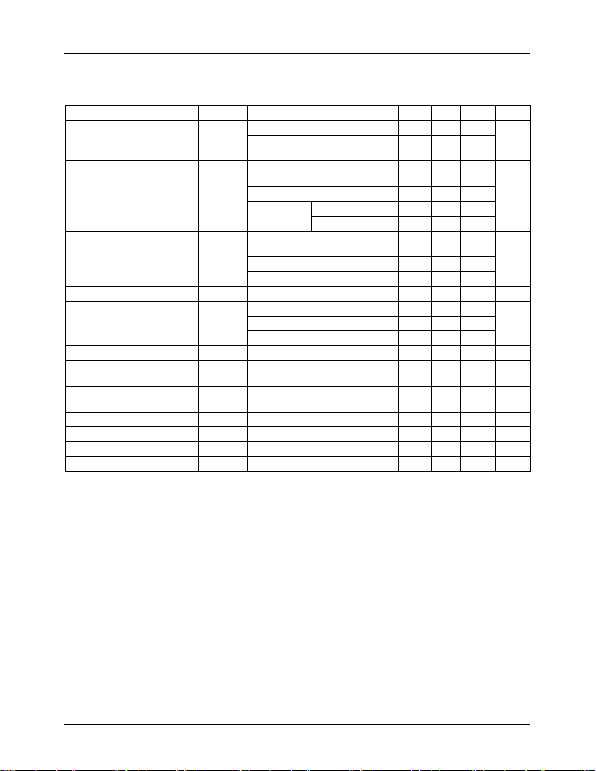MC78XX/LM78XX/MC78XXA
16
Electrical Characteristics (MC7812A)
(Refer to the test circuits. 0°C < TJ < 125°C, Io =1A, V I = 19V, C I=0.33µF, C O=0.1µF, unless otherwise specified)
Note:
1. Load and line regulation are specified at constant junction temperature. Change in VO due to heating effects must be taken
into account separately. Pulse testing with low duty is used.
Parameter Symbol Conditions Min. Typ. Max. Unit
Output Voltage V O TJ =+25 °C 11.75 12 12.25 V
IO = 5mA to 1A, PO 15W
VI = 14.8V to 27V 11.5 12 12.5
Line Regulation (Note1) Regline
VI= 14.8V to 30V
IO = 500mA - 10 120
mV
VI= 16V to 22V - 4 120
TJ =+25 °C VI= 14.5V to 27V - 10 120
VI= 16V to 22V - 3 60
TJ =+25 °C
IO = 5mA to 1.5A - 12 100 mV
IO = 5mA to 1.0A - 12 100
IO = 250mA to 750mA - 5 50
Quiescent Current IQ TJ =+25°C-5.16.0mA
Quiescent Current Change IQ
VI = 15V to 30V, TJ=+25 °C- 0.8
mA VI = 14V to 27V, IO = 500mA - 0.8
IO = 5mA to 1.0A - 0.5
Output Voltage Drift V/T I
O = 5mA - -1.0 - mV/°C
Output Noise Voltage VN f = 10Hz to 100KHz
TA =+25°C-10-µV/Vo
Ripple Rejection RR f = 120Hz, IO = 500mA
VI = 14V to 24V -60- dB
Dropout Voltage VDrop IO = 1A, TJ =+25°C-2.0-V
Output Resistance rO f = 1KHz - 18 - m
Short Circuit Current ISC VI= 35V, TA =+25 °C - 250 - mA
Peak Current IPK TJ=+25 °C-2.2-A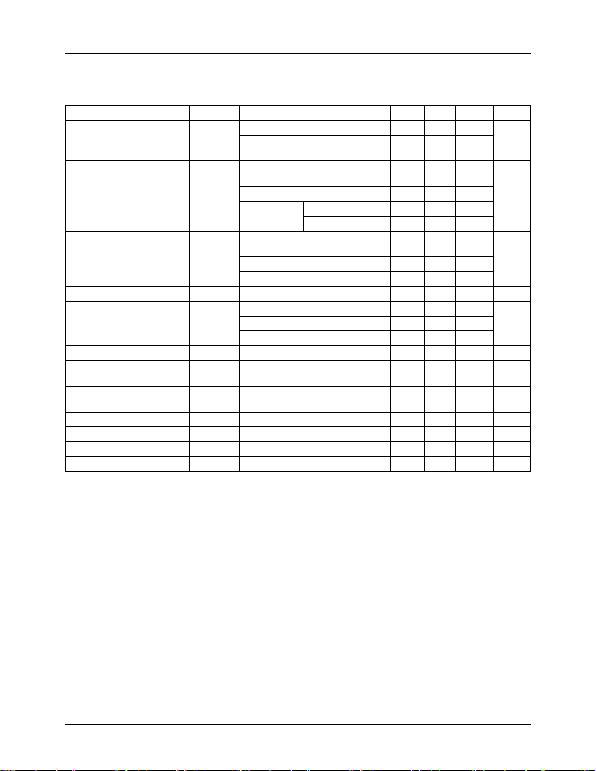MC78XX/LM78XX/MC78XXA
17
Electrical Characteristics (MC7815A)
(Refer to the test circuits. 0°C < TJ < 125°C, Io =1A, V I =23V, C I=0.33µF, C O=0.1µF, unless otherwise specified)
Note:
1. Load and line regulation are specified at constant junction temperature. Change in VO due to heating effects must be taken
into account separately. Pulse testing with low duty is used.
Parameter Symbol Conditions Min. Typ. Max. Unit
Output Voltage VO TJ =+25 °C 14.7 15 15.3 V
IO = 5mA to 1A, PO 15W
VI = 17.7V to 30V 14.4 15 15.6
Line Regulation (Note1) Regline
VI= 17.9V to 30V
IO = 500mA - 10 150
mV
VI= 20V to 26V - 5 150
TJ =+25°C VI= 17.5V to 30V - 11 150
VI= 20V to 26V - 3 75
TJ =+25 °C
IO = 5mA to 1.5A - 12 100 mV
IO = 5mA to 1.0A - 12 100
IO = 250mA to 750mA - 5 50
Quiescent Current IQ TJ =+25 °C-5.26.0mA
Quiescent Current Change IQ
VI = 17.5V to 30V, TJ =+25 °C--0.8
mA VI = 17.5V to 30V, IO = 500mA - - 0.8
IO = 5mA to 1.0A - - 0.5
Output Voltage Drift V/T I
O = 5mA - -1.0 - mV/°C
Output Noise Voltage VN f = 10Hz to 100KHz
TA =+25 °C-10-µV/Vo
Ripple Rejection RR f = 120Hz, IO = 500mA
VI = 18.5V to 28.5V -58- dB
Dropout Voltage VDrop IO = 1A, TJ =+25 °C-2.0-V
Output Resistance rO f = 1KHz - 19 - m
Short Circuit Current ISC VI= 35V, TA =+25 °C - 250 - mA
Peak Current IPK TJ=+25°C-2.2-A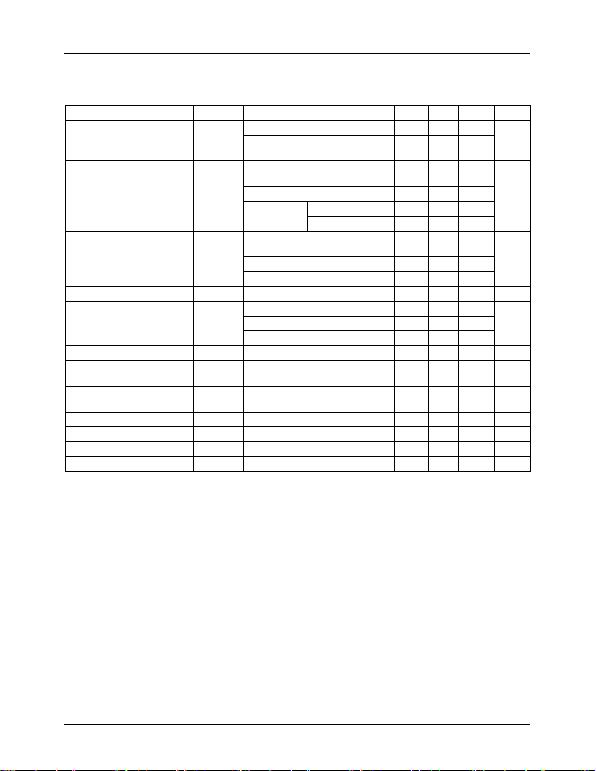MC78XX/LM78XX/MC78XXA
18
Electrical Characteristics (MC7818A)
(Refer to the test circuits. 0°C < TJ < 125°C, Io =1A, V I = 27V, C I=0.33µF, C O=0.1µF, unless otherwise specified)
Note:
1. Load and line regulation are specified at constant junction temperature. Change in VO due to heating effects must be taken
into account separately. Pulse testing with low duty is used.
Parameter Symbol Conditions Min. Typ. Max. Unit
Output Voltage VO TJ =+25 °C 17.64 18 18.36 V
IO = 5mA to 1A, PO 15W
VI = 21V to 33V 17.3 18 18.7
Line Regulation (Note1) Regline
VI= 21V to 33V
IO = 500mA - 15 180
mV
VI= 21V to 33V - 5 180
TJ =+25 °C VI= 20.6V to 33V - 15 180
VI= 24V to 30V - 5 90
TJ =+25°C
IO = 5mA to 1.5A - 15 100 mV
IO = 5mA to 1.0A - 15 100
IO = 250mA to 750mA - 7 50
Quiescent Current IQ TJ =+25 °C-5.26.0mA
Quiescent Current Change IQ
VI = 21V to 33V, TJ=+25 °C--0.8
mA VI = 21V to 33V, IO = 500mA - - 0.8
IO = 5mA to 1.0A - - 0.5
Output Voltage Drift V/T I
O = 5mA - -1.0 - mV/ °C
Output Noise Voltage VN f = 10Hz to 100KHz
TA =+25°C-10-
µV/Vo
Ripple Rejection RR f = 120Hz, IO = 500mA
VI = 22V to 32V -57- dB
Dropout Voltage VDrop IO = 1A, TJ =+25°C-2.0-V
Output Resistance rO f = 1KHz - 19 - m
Short Circuit Current ISC VI= 35V, TA =+25°C - 250 - mA
Peak Current IPK TJ=+25 °C-2.2-A